Courses
Courses for Kids
Free study material
Free LIVE classes
More

# NCERT Solutions for Class 10 Maths Chapter 13 - ExerciseLIVE
Join Vedantu’s FREE Mastercalss

## NCERT Solutions for Class 10 Maths Chapter 13 Surface Areas And Volumes (Ex 13.5) Exercise 13.5

### Important Formulas to Remember to Solve Questions of Exercise 13.5 of NCERT Maths Class 10

Exercise 13.5 Class 10 NCERT Maths is an optional exercise. It deals with the various types of mixed questions related to the volume, curved surface area and total surface area of the cylinder, cone, sphere, cuboid and cube. Some of the questions are related to the frustum of a cone.

Below given are the formulas used in Exercise 13.5 Class 10 NCERT Maths.

• Volume of cylinder = area of cross-section x length = πr2 x l

• Mass = Volume x Density

• Hypotenuse2 = Base2 + Height2

• Area of triangle = 12✖ Base ✖ Height

• Volume of cone =πr2 h3

• Volume of double cone = Volume of upper cone + Volume of lower cone

• Curved surface area of cone = πrl

• Surface area of double cone = Curved surface area of upper cone + Curved surface area of lower cone

• Volume of cuboid = length x breadth x height

• Volume of rain = Area of valley x Rainfall in valley (for question 4)

• Volume of river = length x breadth x depth (for question 4)

Last updated date: 02nd Oct 2023
Total views: 512.4k
Views today: 9.12k
Watch videos on
NCERT Solutions for Class 10 Maths Chapter 13 - ExerciseSurface Areas and Volumes Full Chapter in One Shot | CBSE Class 10 Maths Chap 13 | Term 2 Solutions
Vedantu 9&10
SubscribeShare
8K likes
125.5K Views
1 year ago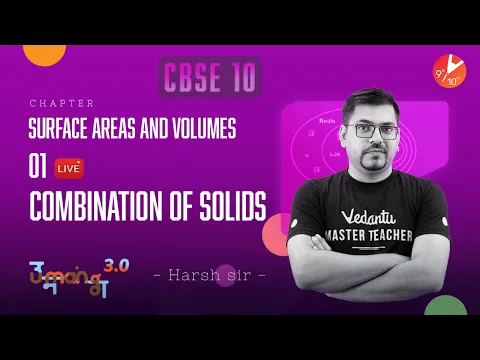Surface Areas and Volumes L-1 [ Combination of Solids ] CBSE Class 10 Maths Ch13 | Term 2 Solutions
Vedantu 9&10
5.9K likes
148.6K Views
1 year ago
Play QuizSurface Area and Volume L1 | Surface Areas and Volumes of Combinations of Solids CBSE Class 10 Maths
Vedantu 9&10
6.9K likes
168.1K Views
3 years ago
Play Quiz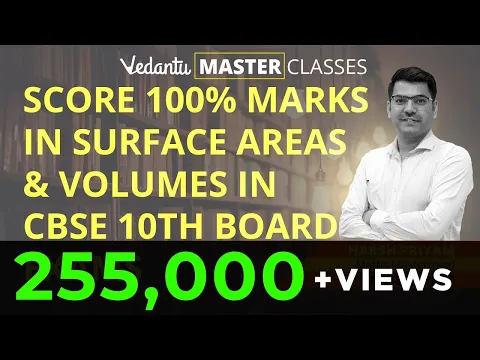Master Surface Area and Volume Class 10 | Surface Area and Volume All Formulas | Frustum of a Cone
Vedantu 9&10
6.8K likes
274.6K Views
4 years ago

## Access NCERT Solutions for Class 10 Maths Chapter 13 – Surface Areas and Volumes

Exercise 13.5

1. A copper wire, 3 mm in diameter, is wound about a cylinder whose length is 12 cm, and diameter 10 cm, so as to cover the curved surface of the cylinder. Find the length and mass of the wire, assuming the density of copper to be 8.88 g per $\text{c}{{\text{m}}^{3}}$.

Ans: Diameter of the copper wire = $3\text{ mm}\approx 0.3\text{ cm}$

So, the radius of the copper wire = $\frac{0.3}{2}\text{ cm = 0}\text{.15 cm}$

Diameter of the cylinder = 10cm.

So, the radius of the cylinder (r)= 5cm.

Length of the cylinder = 12cm.

One round of wire around the cylinder covers 3mm height of the cylinder.

Total number of rounds = $\frac{\text{Total height of the cylinder}}{\text{Diameter of wire}}$

$\Rightarrow \frac{12}{\text{0}\text{.3}}\text{ = 40 rounds}$

Length of wire in one complete round = $2\pi r$

$=2\pi \times 5$

$=10\pi \text{ }cm$

Hence, length of wire in 40 rounds (l) =  $40\times 10\pi \text{ }cm$

$=1256\text{ cm}$

Volume of wire = area of cross section of wire * length

$=\text{ }\pi {{r}^{2}}\times l$

$=\text{ 3}\text{.14}\times {{(0.15)}^{2}}\times 1256$

$=\text{ }88.898\text{ c}{{\text{m}}^{3}}$

Mass of the wire = volume of wire * density

$=\text{ }88.898\times 8.88$

$=\text{ }789.41\text{gm}$

The length of the wire $=1256\text{ cm}$ and mass of the wire $=\text{ }789.41\text{gm}$.

2. A right triangle whose sides are 3 cm and 4 cm (other than hypotenuse) is made to revolve about its hypotenuse. Find the volume and surface area of the double cone so formed. (Choose value of $\text{ }\!\!\pi\!\!\text{ }$ as found appropriate.)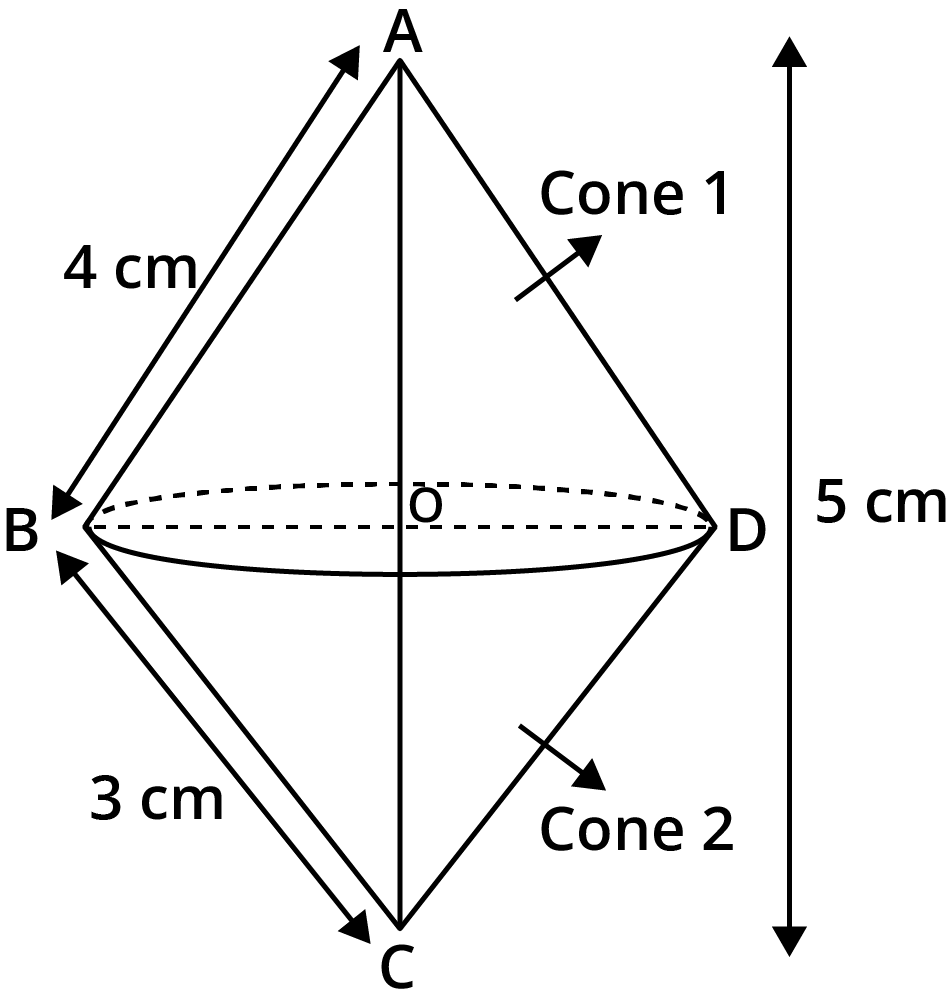Ans: Two sides of the right triangle , base (b) = 3cm and length (l) = 4cm.

Hypotenuse of the right triangle using Pythagoras theorem: $\sqrt{{{b}^{2}}+{{l}^{2}}}$

=$\sqrt{{{3}^{2}}+{{4}^{2}}}$

=$5\text{ cm}$

Two cone is formed because of revolution of right triangle about its hypotenuse.

Area of $\Delta ABC$ = $\frac{1}{2}\times l\times b$

$\Rightarrow \frac{1}{2}\times AC\times OB=\frac{1}{2}\times 4\times 3$

$\Rightarrow \frac{1}{2}\times 5\times OB=6\text{ c}{{\text{m}}^{2}}$

$\Rightarrow OB=\frac{12}{5}cm$

Volume of double cone  = volume of upper cone + volume of lower cone

$=\frac{\pi }{3}\times B{{O}^{2}}\times A\text{O }+\text{ }\frac{\pi }{3}\times B{{O}^{2}}\times C\text{O}$

$=\frac{\pi }{3}\times B{{O}^{2}}\times (A\text{O }+C\text{O)}$

$=\frac{\pi }{3}\times B{{O}^{2}}\times AC$ (AO+CO=AC)

$=\frac{1}{3}\times \frac{22}{7}\times {{\left( \frac{12}{5} \right)}^{2}}\times 5$

$=30.14\text{ c}{{\text{m}}^{3}}$

Surface area of the double cone = Surface area of upper cone + Surface area of lower cone

$=\pi rl\text{ + }\pi rb$

$=\pi \frac{12}{5}\times \text{4 + }\pi \frac{12}{5}\times 3$

$=\pi \left( \frac{36}{5}+\frac{48}{5} \right)$

$=\frac{22}{7}\left( \frac{84}{5} \right)$

$=52.75\text{ c}{{\text{m}}^{2}}$

Volume of double cone $=30.14\text{ c}{{\text{m}}^{3}}$

Surface area of the double cone $=52.75\text{ c}{{\text{m}}^{2}}$

3. A cistern, internally measuring $\text{150 cm }\!\!\times\!\!\text{ 120 cm }\!\!\times\!\!\text{ 110 cm}$, has $\text{129600c}{{\text{m}}^{\text{3}}}$ of water in it. Porous bricks are placed in the water until the cistern is full to the brim. Each brick absorbs one-seventeenth of its own volume of water. How many bricks can be put in without overflowing the water, each brick being $\text{22}\text{.5 cm }\!\!\times\!\!\text{ 7}\text{.5 cm }\!\!\times\!\!\text{ 6}\text{.5 cm}$?

Ans: Dimension of cistern = $150\text{cm }\times \text{ 120cm }\times \text{ 110cm}$

So, the volume of cistern = $1980000\text{c}{{\text{m}}^{3}}$.

Volume of water = $129600\text{c}{{\text{m}}^{3}}$.

Volume of cistern filled = $1980000\text{c}{{\text{m}}^{3}}\text{ - }129600\text{c}{{\text{m}}^{3}}$

$=1850400\text{c}{{\text{m}}^{3}}$

Dimension of bricks = $22.5\text{cm }\times \text{ 7}\text{.5cm }\times \text{ 6}\text{.5cm}$

Volume of bricks = $1096.875\text{c}{{\text{m}}^{3}}$

Let the number of porous bricks placed in cistern be n.

Volume of n bricks = n * Volume of brick

$=n\times 1096.875\text{c}{{\text{m}}^{3}}$

It is given that each brick absorbs one-seventeenth of its own volume.

Then, the volume absorbed by n bricks =  $\frac{n\times 1096.875}{17}\text{c}{{\text{m}}^{3}}$.

According to the question,

The volume of n bricks = volume absorbed by n bricks + Volume to be filled in cistern

$\Rightarrow n\times 1096.875\text{ = }\frac{n\times 1096.875}{17}\text{ + 1850400 }$

$\Rightarrow \frac{16n\times 1096.875}{17}\text{ = 1850400 }$

$\Rightarrow n\text{ = 1792}\text{.41 }$

Therefore, the number of required bricks = 1792.

4. In one fortnight of a given month, there was a rainfall of 10 cm in a river valley .If the area of the valley is $\text{7280K}{{\text{m}}^{\text{2}}}$, show that the total rainfall was approximately equivalent to the addition to the normal water of three rivers each 1072 Km long ,75 m wide and 3 m deep.

Ans: The area of valley = $\text{7280K}{{\text{m}}^{\text{2}}}$.

Rainfall in valley = $10cm\text{ }\approx \text{ }\frac{10}{100\times 1000}km$

Volume of rain = $\frac{\text{7280}\times \text{10}}{100\times 1000}k{{m}^{3}}$

$=0.728k{{m}^{3}}$

Length of the river = 1072km

Breadth of the river = $75m\text{ }\approx \text{ 0}\text{.075}km$

Depth of the river = $3m\text{ }\approx \text{ 0}\text{.003}km$

Volume of a river = $\text{(1072}\times \text{0}\text{.075}\times \text{0}\text{.003)}k{{m}^{3}}$

$=0.2412\text{ }k{{m}^{3}}$

Volume of three river $=3\times 0.2412\text{ }k{{m}^{3}}$

$=0.7236\text{ }k{{m}^{3}}$

Hence,the total rainfall was approximately equivalent to the addition to the normal water of three rivers.

5. An oil funnel made of tin sheet consists of a 10 cm long cylindrical portion attached to a frustum of a cone. If the total height is 22 cm, diameter of the cylindrical portion is 8 cm and the diameter of the top of the funnel is 18 cm, find the area of the tin sheet required to make the funnel (see the given figure).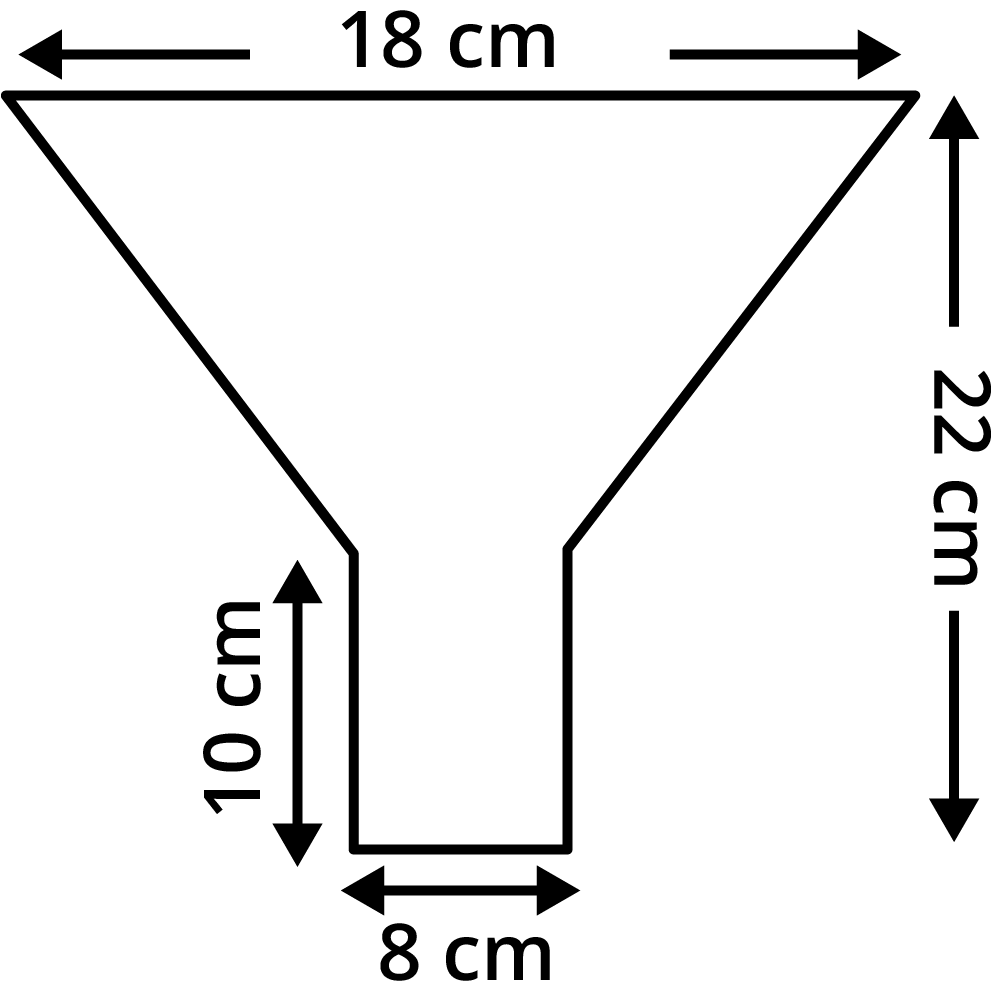Ans: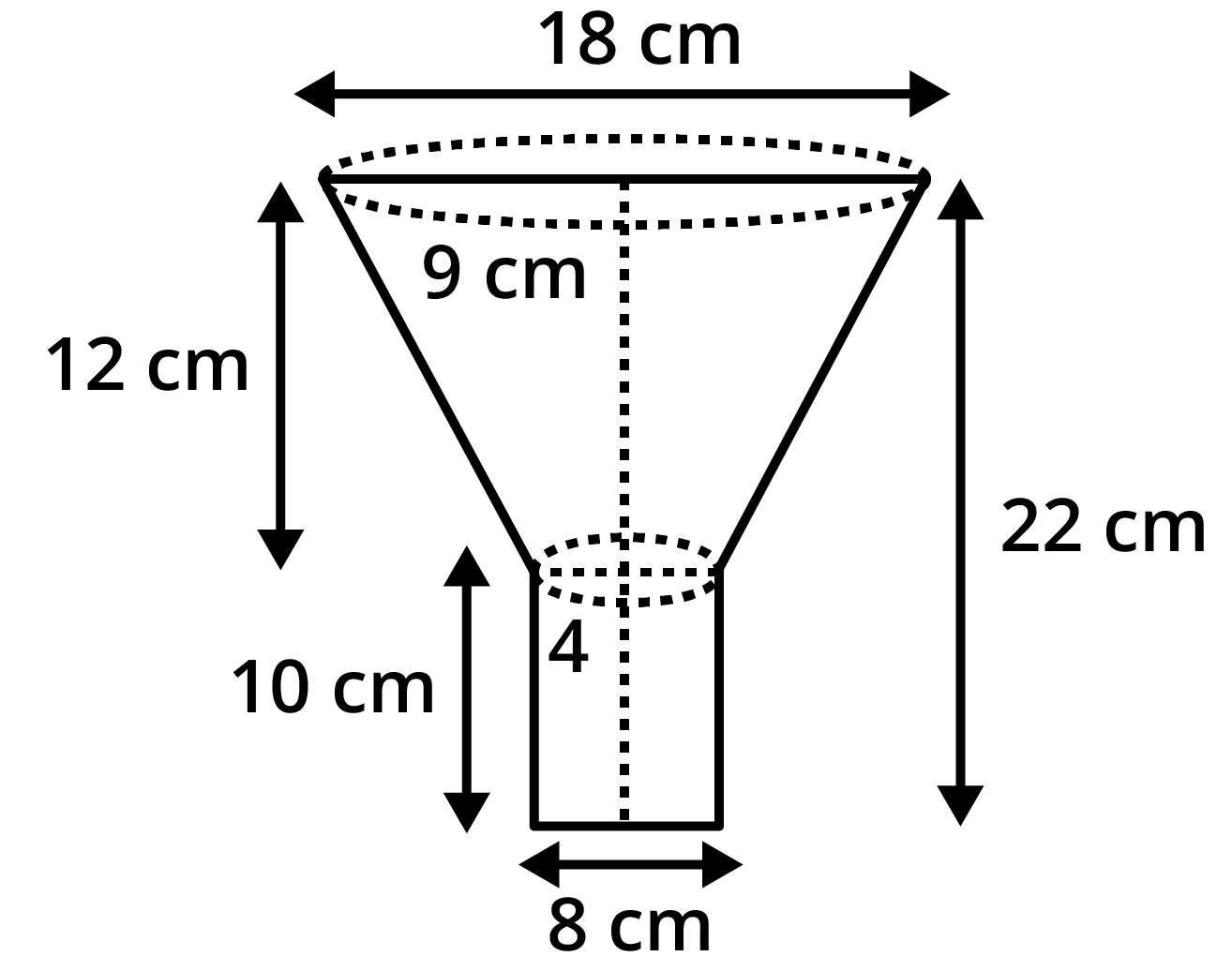Height of the cylinder = 10cm.

Total height = 22cm.

Height of the frustum (h) = Total height  - Height of the cylinder

$=(22-10)cm$

$=12cm$

Diameter of lower base = 8cm.

Diameter of top funnel = 18cm.

Therefore, the radius (R) = 9cm.

Slant height of the frustum (l) =  $\sqrt{{{(R-r)}^{2}}+{{h}^{2}}}$

$=\sqrt{{{(9-4)}^{2}}+{{12}^{2}}}$

$=\sqrt{{{5}^{2}}+{{12}^{2}}}$

$=\sqrt{25+144}$

$=\sqrt{169}$

$=13cm$

Area of thin sheet which is required = curved surface area of frustum + curved surface area of cylinder.

$=\pi (r+R)l\text{ + 2}\pi \text{rh}$

$=\frac{22}{7}\times (4+9)\times 13\text{ + 2}\times \frac{22}{7}\times 4\times 10$

$=\frac{22}{7}\times 249$

$=782.57\text{ c}{{\text{m}}^{2}}$

6. Derive the formula for the curved surface area and total surface area of the frustum of a cone, given to you in Section 13.5, using the symbols as explained.

Ans: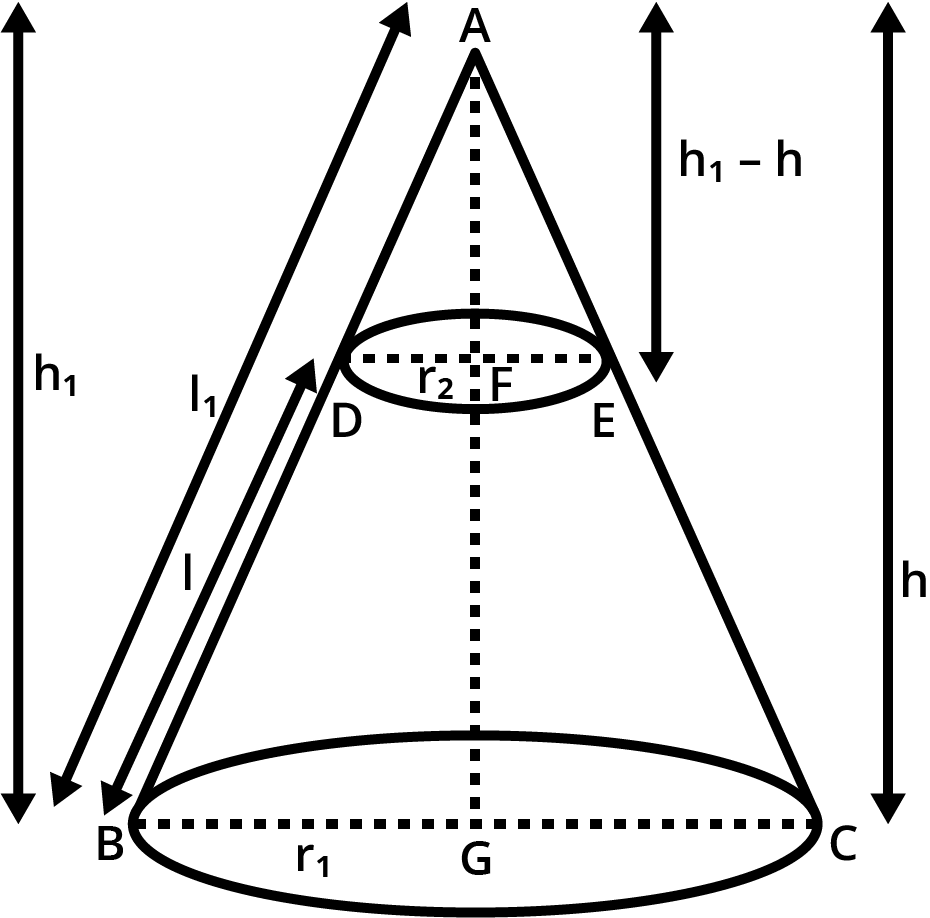Let ABC be a cone of height ${{h}_{1}}$ and slant height of ${{l}_{1}}$.The radius of base is ${{r}_{1}}$.

From the cone ABC a frustum DECB is cut by a plane parallel to its base. The height of frustum is  h and slant height is l. The radius of upper end is ${{r}_{2}}$.

Considering the $\Delta ABG$ and $\Delta ADF$,

$BG\parallel DF$ and $\angle A$ is common in both . Hence, $\Delta ABG\sim \Delta ADF$.

Therefore, $\frac{DF}{BG}=\frac{AF}{AG}=\frac{AD}{AB}$

$\Rightarrow \frac{{{r}_{2}}}{{{r}_{1}}}=\frac{{{h}_{1}}-h}{{{h}_{1}}}=\frac{{{l}_{1}}-l}{{{l}_{1}}}$

$\Rightarrow \frac{\text{l}}{{{l}_{1}}}=1-\frac{{{r}_{2}}}{{{r}_{1}}}$

After rearranging,

$\Rightarrow {{l}_{1}}=\frac{{{r}_{1}}l}{{{r}_{1}}-{{r}_{2}}}$

Curve surface area of frustum DECB = Curve surface area of cone ABC - Curve surface area of ADE.

$=\pi {{r}_{1}}{{l}_{1}}\text{ - }\pi {{r}_{2}}({{l}_{1}}-l)$

$=\pi {{r}_{1}}\left( \frac{{{r}_{1}}l}{{{r}_{1}}-{{r}_{2}}} \right)\text{ - }\pi {{r}_{2}}\left[ \left( \frac{{{r}_{1}}l}{{{r}_{1}}-{{r}_{2}}} \right)-l \right]$

$=\frac{\pi {{r}_{1}}^{2}l}{{{r}_{1}}-{{r}_{2}}}\text{ - }\pi {{r}_{2}}\left( \frac{{{r}_{1}}l-{{r}_{1}}l+{{r}_{2}}l}{{{r}_{1}}-{{r}_{2}}} \right)$

$=\frac{\pi {{r}_{1}}^{2}l}{{{r}_{1}}-{{r}_{2}}}\text{ - }\frac{\pi {{r}_{2}}^{2}l}{{{r}_{1}}-{{r}_{2}}}$

$=\pi l\left( \frac{{{r}_{1}}^{2}-{{r}_{2}}^{2}}{{{r}_{1}}-{{r}_{2}}}\text{ } \right)$

$=\pi l\left( \frac{\left( {{r}_{1}}+{{r}_{2}} \right)\left( {{r}_{1}}-{{r}_{2}} \right)}{{{r}_{1}}-{{r}_{2}}}\text{ } \right)$

Curve surface area of frustum $=\pi \left( {{r}_{1}}+{{r}_{2}} \right)l$

The total surface area of frustum = Curve surface area of frustum + Area of upper circular end + Area of the lower circular end

$=\pi \left( {{r}_{1}}+{{r}_{2}} \right)l\text{ }+\text{ }\pi {{r}_{2}}^{2}\text{ + }\pi {{r}_{1}}^{2}$

The total surface area of frustum $=\pi \left[ \left( {{r}_{1}}+{{r}_{2}} \right)l\text{ }+\text{ }{{r}_{2}}^{2}\text{ + }{{r}_{1}}^{2} \right]$.

7. Derive the formula for the volume of the frustum of a cone, given to you in Section 13.5, using the symbols as explained.

Ans: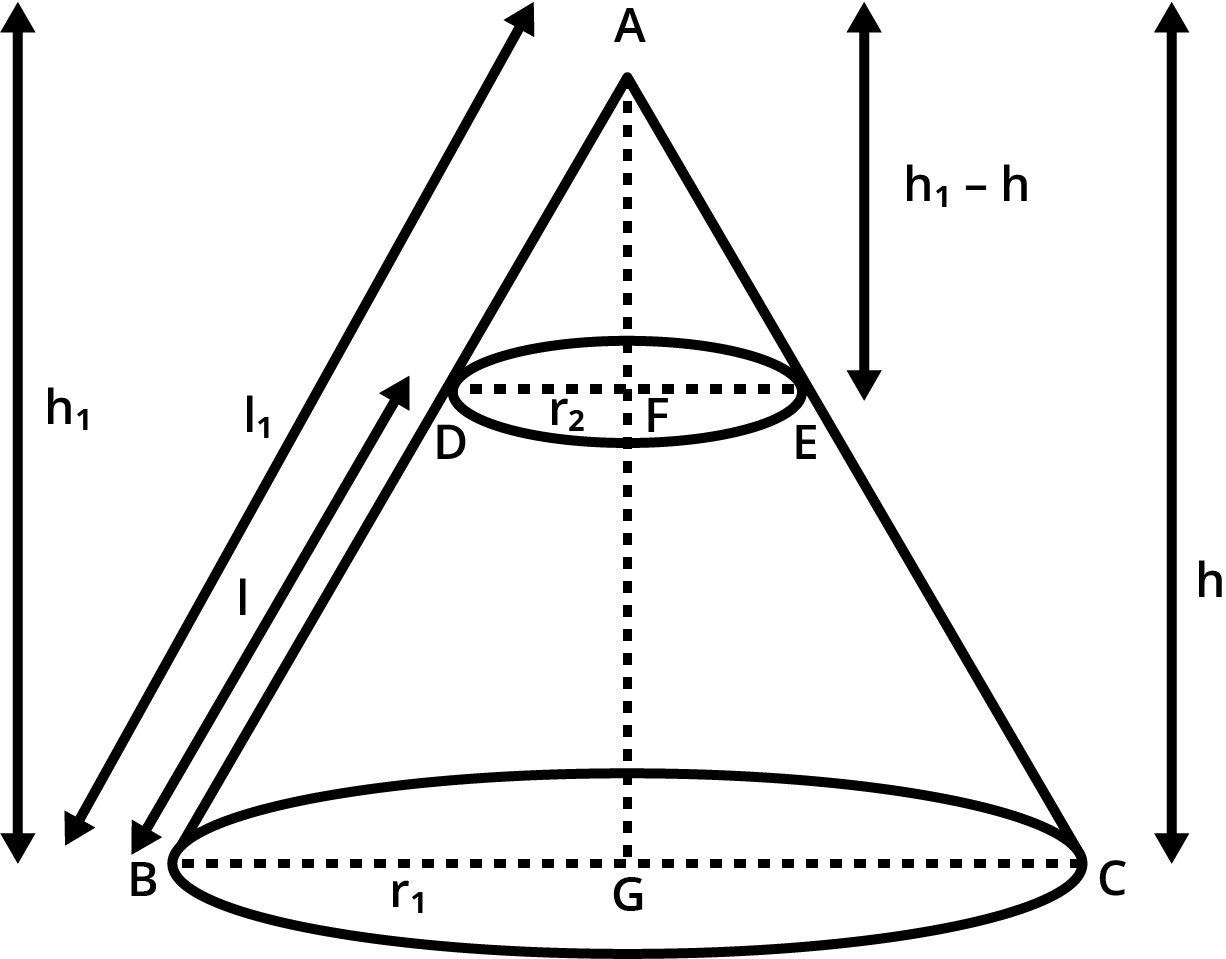Let ABC be a cone of height ${{h}_{1}}$ and slant height of ${{l}_{1}}$.The radius of base is ${{r}_{1}}$.

From the cone ABC a frustum DECB is cut by a plane parallel to its base. The height of frustum is  h and slant height is l. The radius of upper end is ${{r}_{2}}$.

Considering the $\Delta ABG$ and $\Delta ADF$,

$BG\parallel DF$ and $\angle A$ is common in both triangle. Hence, $\Delta ABG\sim \Delta ADF$.

Therefore, $\frac{DF}{BG}=\frac{AF}{AG}=\frac{AD}{AB}$

$\Rightarrow \frac{{{r}_{2}}}{{{r}_{1}}}=\frac{{{h}_{1}}-h}{{{h}_{1}}}=\frac{{{l}_{1}}-l}{{{l}_{1}}}$

$\Rightarrow \frac{h}{{{h}_{1}}}=1-\frac{{{r}_{2}}}{{{r}_{1}}}$

After rearranging,

$\Rightarrow {{h}_{1}}=\frac{{{r}_{1}}h}{{{r}_{1}}-{{r}_{2}}}$

The total volume of frustum of the cone  = Volume of cone ABC - Volume of cone ADE

$=\frac{1}{3}\pi {{({{r}_{1}})}^{2}}{{h}_{1}}\text{ - }\frac{1}{3}\pi ({{r}_{2}})^2({{h}_{1}}-h)$

$=\left( \frac{\pi }{3} \right)\left[ {{({{r}_{1}})}^{2}}{{h}_{1}}\text{- }{{r}_{2}}^{2}({{h}_{1}}-h) \right]$

$=\left( \frac{\pi }{3} \right)\left[ {{r}_{1}}^{2}\left( \frac{h{{r}_{1}}}{{{r}_{1}}-{{r}_{2}}} \right)\text{- }{{r}_{2}}^{2}\left( \frac{h{{r}_{1}}}{{{r}_{1}}-{{r}_{2}}}-h \right) \right]$

$=\left( \frac{\pi }{3} \right)\left[ \left( \frac{h{{r}_{1}}^{3}}{{{r}_{1}}-{{r}_{2}}} \right)\text{- }{{r}_{2}}^{2}\left( \frac{h{{r}_{1}}-h{{r}_{1}}+h{{r}_{2}}}{{{r}_{1}}-{{r}_{2}}} \right) \right]$

$=\left( \frac{\pi }{3} \right)\left( \frac{h{{r}_{1}}^{3}}{{{r}_{1}}-{{r}_{2}}}-\frac{h{{r}_{2}}^{3}}{{{r}_{1}}-{{r}_{2}}} \right)$

$=\left( \frac{\pi }{3}h \right)\left( \frac{{{r}_{1}}^{3}-{{r}_{2}}^{3}}{{{r}_{1}}-{{r}_{2}}} \right)$

As we all know ${{a}^{3}}-{{b}^{3}}=(a-b)({{a}^{2}}+{{b}^{2}}+ab)$

$=\left( \frac{\pi }{3}h \right)\left( \frac{({{r}_{1}}-{{r}_{2}})({{r}_{1}}^{2}+{{r}_{2}}^{2}+{{r}_{1}}{{r}_{2}})}{{{r}_{1}}-{{r}_{2}}} \right)$

$=\left( \frac{\pi }{3}h \right)({{r}_{1}}^{2}+{{r}_{2}}^{2}+{{r}_{1}}{{r}_{2}})$

So, Volume of frustum of the cone = $\frac{1}{3}\pi h({{r}_{1}}^{2}+{{r}_{2}}^{2}+{{r}_{1}}{{r}_{2}})$

## NCERT Solutions for Class 10 Maths Chapter 13 Surface Areas and Volumes Exercise 13.5

Opting for the NCERT solutions for Ex 13.5 Class 10 Maths is considered as the best option for the CBSE students when it comes to exam preparation. This chapter consists of many exercises. Out of which we have provided the Exercise 13.5 Class 10 Maths NCERT solutions on this page in PDF format. You can download this solution as per your convenience or you can study it directly from our website/ app online.

Vedantu in-house subject matter experts have solved the problems/ questions from the exercise with the utmost care and by following all the guidelines by CBSE. Class 10 students who are thorough with all the concepts from the Subject Surface Areas and Volumes textbook and quite well-versed with all the problems from the exercises given in it, then any student can easily score the highest possible marks in the final exam. With the help of this Class 10 Maths Chapter 13 Exercise 13.5 solutions, students can easily understand the pattern of questions that can be asked in the exam from this chapter and also learn the marks weightage of the chapter. So that they can prepare themselves accordingly for the final exam.

Besides these NCERT solutions for Class 10 Maths Chapter 13 Exercise 13.5, there are plenty of exercises in this chapter which contain innumerable questions as well. All these questions are solved/answered by our in-house subject experts as mentioned earlier. Hence all of these are bound to be of superior quality and anyone can refer to these during the time of exam preparation. In order to score the best possible marks in the class, it is really important to understand all the concepts of the textbooks and solve the problems from the exercises given next to it.

Do not delay any more. Download the NCERT solutions for Class 10 Maths Chapter 13 Exercise 13.5 from Vedantu website now for better exam preparation. If you have the Vedantu app in your phone, you can download the same through the app as well. The best part of these solutions is these can be accessed both online and offline as well.

### NCERT Solutions for Class 10 Maths Chapter 13 Other Exercises

 Chapter 13 Surface Areas and Volumes All Exercises in PDF Format Exercise 13.1 9 Questions and Solutions Exercise 13.2 8 Questions and Solutions Exercise 13.3 9 Questions and Solutions Exercise 13.4 5 Questions and Solutions

## FAQs on NCERT Solutions for Class 10 Maths Chapter 13 - Exercise

1. How many questions are there in exercise 13.5 of Class 10th Maths Chapter 13?

Exercise 13.5 of NCERT Class 10 Maths Chapter 10 has a total of seven questions and even though it is an optional exercise, students are advised to attempt it and get their doubts clarified by their mentors or teachers. These questions are important and can be asked in your exams as well. The concepts covered in this exercise are in adherence to the contents of Chapter 13.

2. Is Exercise 13.5 (Chapter 13) of Class 10th Maths difficult?

The difficulty will depend on how well the student has grasped the fundamentals and has the ability to efficiently implement and apply those fundamentals in solving the problems and questions. These questions are very important and hence must not be skipped even though it is an optional exercise. Practice well and visit the page NCERT Solutions Class 10 Maths Chapter 13 Exercise 13.5 on the official website of Vedantu or download the Vedantu app for multiple modules regarding this exercise and also to other exercises of this chapter at free of cost.

3. What is Surface Area in Class 10?

As a 2-dimensional notion, Surface Area is measured in metres, centimetres, or millimetres (mm), while Volume is measured in metres, centimetres, and millimetres (mm). Circular, square, or triangle 2-D shapes can be measured in surface area, but they cannot be measured in volume. A surface area is the area or region of an object's surface.

4. What do you mean by Area and Volume?

There are two types of objects: two-dimensional areas and three three-dimensional objects, such as areas and volumes. When it comes to volume, it's a good number. In the same way, that area is the outward space, volume is the interior capacity of the body. The area is measured in square units, whereas volume is measured in cubic inches.

5. What does Curved Surface Area mean in Class 10?

Only the curved section of a 3D figure is included in the curved surface area calculation. It is generally accepted that lateral surface area and curved surface area have the same meaning in terms of their definitions. A cuboid's surface area is calculated using the lateral surface area whereas the cylindrical surface area is calculated by subtracting the top and bottom surfaces.